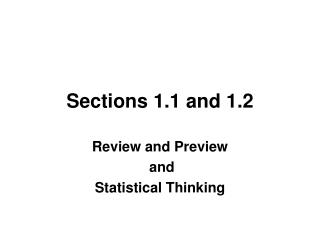# Sections 1.1 and 1.2 - PowerPoint PPT PresentationDownload PresentationSections 1.1 and 1.2

Sections 1.1 and 1.2
Download Presentation## Sections 1.1 and 1.2

- - - - - - - - - - - - - - - - - - - - - - - - - - - E N D - - - - - - - - - - - - - - - - - - - - - - - - - - -
##### Presentation Transcript

1. Sections 1.1 and 1.2 Review and Preview and Statistical Thinking

2. WHAT IS STATISTICS? • Actual and specific numbers derived from data. • Definition: A collection of methods for planning experiments, obtaining data, and then organizing, summarizing, presenting, analyzing, interpreting, and drawing conclusions based on the data.

3. SOME IMPORTANT DEFINITIONS • Population: the entire group of subjects or complete collection of measurements to be studied. • Census: the collection of data from every element in a population. • Sample: a subset of, or sub-collection, from a population. • Parameter: a numerical measurement describing a characteristic or attribute of a population. • Statistic: a numerical measurement describing a characteristic or attribute of a sample.

4. STATISTICAL THINKING This section introduces basic principles of statistical thinking used throughout this book. Whether conducting statistical analysis of data that we have collected, or analyzing a statistical analysis done by someone else, we should not rely on blind acceptance of mathematical calculation. We should consider these factors: • Context of Data • Source of the Data • Sampling Method • Conclusions • Practical Implications

5. CONTEXT • What do the values represent? • Where did the data come from? • Why were they collected? • An understanding of the context will directly affect the statistical procedure used.

6. SOURCE OF DATA • Is the source objective? • Is the source biased? • Is there some incentive to distort or spin results to support some self-serving position? • Is there something to gain or lose by distorting results? • Be vigilant and skeptical of studies from sources that may be biased.

7. SAMPLING METHOD • Does the method chosen greatly influence the validity of the conclusion? • Voluntary response (or self-selected) samples often have bias (those with special interest are more likely to participate). These samples’ results are not necessarily valid. • Other methods are more likely to produce good results.

8. CONCLUSIONS • Make statements that are clear to those without an understanding of statistics and its terminology. • Avoid making statements not justified by the statistical analysis.

9. PRACTICAL IMPLICATIONS • State practical implications of the results. • There may exist some statistical significanceyet there may be NOpractical significance. • Common sense might suggest that the finding does not make enough of a difference to justify its use or to be practical.

10. STATISTICAL SIGNIFICANCE • Consider the likelihood of getting the results by chance. • If results could easily occur by chance, then they are not statistically significant. • If the likelihood of getting the results is so small, then the results are statistically significant.